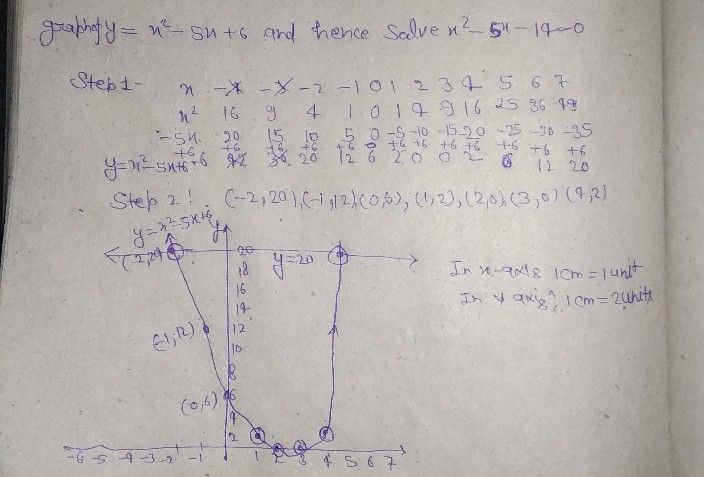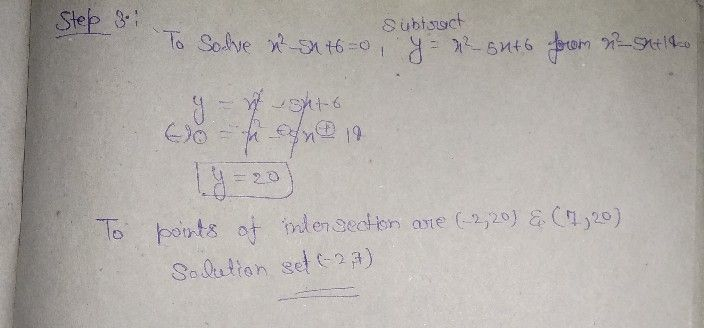Symbol
Problem$1$ Draw the graph of $y=x^{2}-5x-6$ and hence solve $x^{2}-5x-14=0$
10th-13th grade
Geometry
Search count: 105
SolutionQanda teacher - Deepakplease give me review by 5 ?